﻿ 模糊粒子群优化算法的第四方物流运输时间优化
«上一篇文章快速检索 高级检索

 智能系统学报2021, Vol. 16Issue (3): 474-483  DOI: 10.11992/tis.2020040320

### 引用本文LU Fuqiang, LIU Ting, DU Zichao, et al. Convergence fuzzy particle swarm optimization based transportation time optimization of 4PL[J]. CAAI Transactions on Intelligent Systems, 2021, 16(3): 474-483. DOI: 10.11992/tis.202004032.### 文章历史

1. 燕山大学 经济管理学院，河北 秦皇岛 066004;
2. 东北大学 信息科学与工程学院，辽宁 沈阳 816819

Convergence fuzzy particle swarm optimization based transportation time optimization of 4PL
LU Fuqiang 1, LIU Ting 2, DU Zichao 2, BI Hualing 1, HUANG Min 2
1. School of Economics and Management, Yanshan University, Qinhuangdao 066004, China;
2. Faculty of Information Science and Engineering, Northeastern University, Shenyang 816819, China
Abstract: This paper proposed a transportation time optimization model that employed a convergence factor and the convergence fuzzy particle swarm optimization (CFPSO) of membership functions to determine transportation routes and third party agents in the fourth-party logistics (4PL). Three examples with different scales were designed in the simulation experiment. The experimental result of the CFPSO was analyzed and compared with those of the enumeration algorithm, basic particle swarm optimization algorithm, genetic algorithm, and quantum-behaved particle swarm optimization to establish the validity of the proposed model and algorithm.
Key words: fourth party logistics    transportation cost    transportation time    third party online agents    convergence factor    degree of membership function    convergence fuzzy optimization    particle swarm optimization

4PL具有明显的优势，在物流领域中起到举足轻重的作用，得到了国内外学者的广泛研究。同时，4PL也面临着巨大挑战，归结起来可分为2个方面：1)黄敏等提出4PL如何激励3PL提高物流服务质量并降低配送过程中的风险问题；2)如何对资源进一步整合优化使得3PL在可以接受的配送成本条件下提高物流服务质量，使得3PL切实感受到4PL的加入可以使它们能够将自身资源用于其核心业务。因此，考虑在委托商可接受的最大成本约束下，提高加入4PL后的3PL的物流服务质量具有重要研究价值。本文对4PL的路径优化问题进行研究，缩短配送时间，提高物流服务质量。关于4PL的运输路径优化问题，综合考虑3PL的能力、信誉、转运时间及运输成本等因素的影响，如黄敏等充分考虑不确定环境的影响，将3PL代理商的配送时间设定为不确定变量，建立了4PL路径规划模型，并采用多种改进的遗传算法进行求解；崔研等考虑中转发车时间，以运输时间为约束条件，以总成本最小为目标函数，建立了基于一点到多点的多任务第四方物流路径优化模型，并设计蚁群优化算法求解模型；薄桂华等将嵌入删除算法和声搜索算法两阶段算法，用于加快求解带时间窗约束的4PL路径优化问题的运行时间；王勇等考虑了时间和风险因素的约束，研究多任务、多代理商的4PL作业整合优化，采用柔性禁忌算法求解模型；崔研等结合模糊的转运时间和最小的成本约束，研究多点到单点的4PL路径优化问题，设计文化基因算法求解模型；陈建清等建立了赋予多维权的有向图模型，描述第四方物流中路径优化、3PL代理商选择等问题，并提出基于Dijkstra算法的求解方法。对于4PL的路径优化问题，既要考虑委托商提出的成本约束，又要满足客户对运输时间的期望。同时，由于运输环境的不确定性，各个3PL代理商运输能力的不同，城市节点中存在的转运时间，4PL路径优化已然成为一个复杂、影响因素相互制约的系统工程问题。在一定的成本预算约束下，如何设计运输路径，选择合适的3PL代理商，形成一套完整的供应链解决方案以实现客户期望的时间，已经成为4PL亟待解决、不断优化的问题。

1 第四方物流运输时间优化问题 1.1 第四方物流运输问题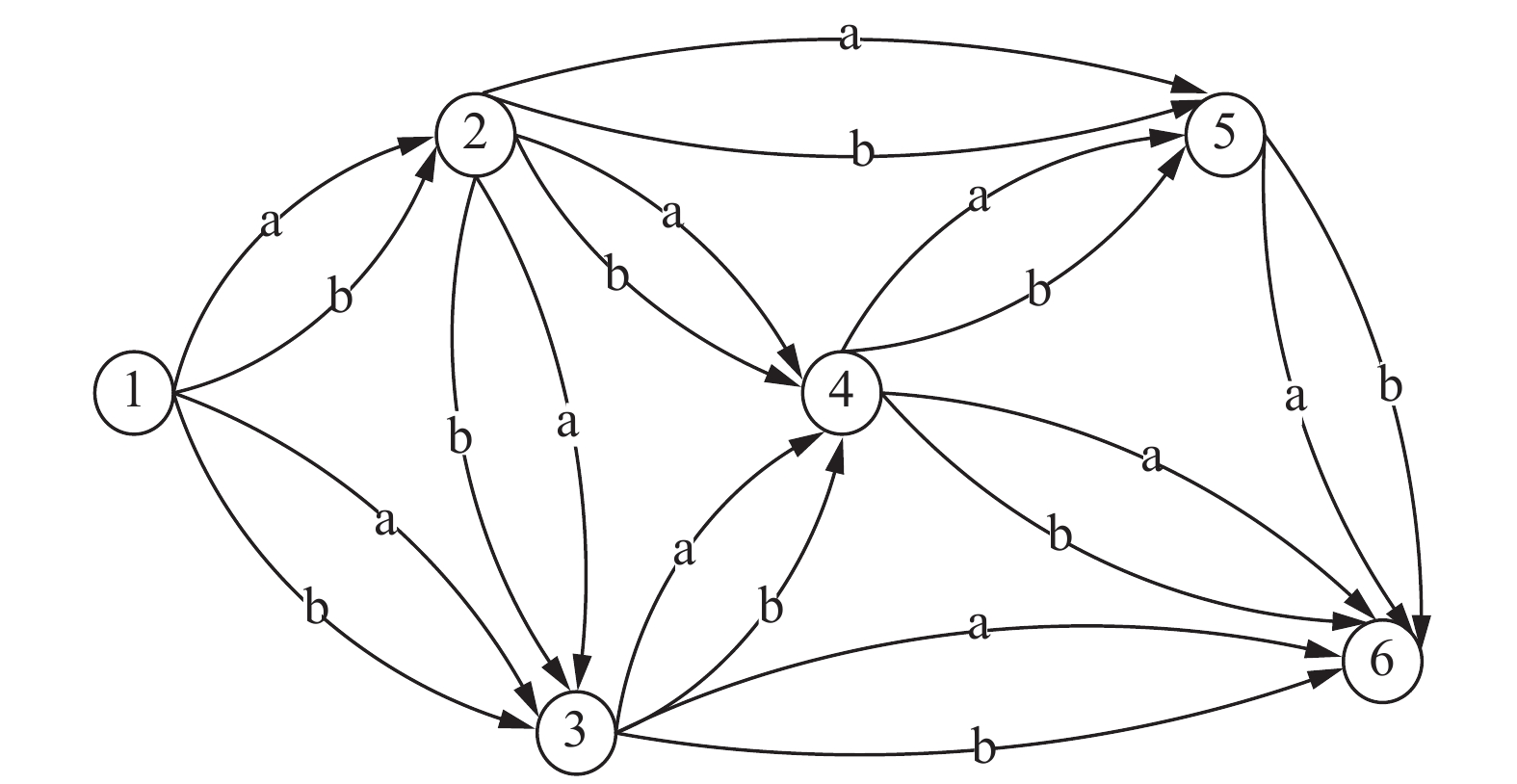Download: 图 1 运输路线网络 Fig. 1 Transport route network

1.2 运输时间优化模型 1.2.1 模型假设与符号定义

1)模型假设

①每个作业任务至多由一个代理商负责完成。

②本问题是单任务问题，且只有一个起点和一个终点。

③从出发点运送到终点的过程中，任务需要完成的总运输量不会改变。

2)符号定义

$x_{ij}^g$ ：作业在节点 $i$ 与节点 $j$ 之间选择代理商 $g$ 完成。

$Q$ ：从配送起点到配送终点需要被配送的总运量。

${Q_g}$ ：代理商 $g$ 能够承担的最大运量。

${d_{ij}}$ ：节点 $i$ 与节点 $j$ 之间的距离。

$c_{ij}^g$ ：代理商 $g$ 在节点 $i$ 与节点 $j$ 之间单位运输距离、单位运载量的运输成本。

$t_{ij}^g$ ：代理商 $g$ 在节点 $i$ 与节点 $j$ 之间运输单位运量货物的运输时间。

$C$ ：委托人能够承担的最大运输成本。

$G$ ：代理商的个数。

$N$ ：网络中的节点数量。

1.2.2 数学模型
 ${{\rm{Min}}}\;\;\mathop \sum \limits_{{{g}} = 1}^G \mathop \sum \limits_{i = 1}^N \mathop \sum \limits_{j = 1}^N t_{ij}^gx_{ij}^g$ (1)
 ${{\rm{s}}{\rm{.t}}.}\;\;\mathop \sum \limits_{g = 1}^G \mathop \sum \limits_{i = 1}^N \mathop \sum \limits_{j = 1}^N {d_{ij}}c_{ij}^gQx_{ij}^g \leqslant C$ (2)
 $\begin{array}{c} {\displaystyle\sum_{j = 1}^N} x_{ij}^g \leqslant 1,i \in \left\{ {N - \left\{ 1 \right\}} \right\}, j \in \left\{ {1,2, \cdots ,N} \right\},\\ g \in \left\{ {1,2, \cdots ,G} \right\} \end{array}$ (3)
 $Q \leqslant {Q_g},g \in \left\{ {1,2, \cdots ,G} \right\}$ (4)
 $\left\{ {\begin{array}{*{20}{l}} {1,\;\;\;\;\;{\text{作业在节点}}i{\text{与}}j{\text{之间选代理商}}g}\\ {0,\;\;\;\;\;{\text{作业在节点}}i{\text{与}}j{\text{之间未选代理商}}} \end{array}} \right.$ (5)

2 收敛模糊粒子群优化算法

4PL运输时间优化问题的自变量众多，且对算法的运行时间和运行结果的精度要求高，因此采用PSO算法进行求解。PSO算法是一种模拟自然界鸟类觅食过程的生物启发式算法[12-13]。PSO初始化为一群随机粒子，这些粒子在搜索空间中4处移动来寻找问题最佳的解。Shi等引入惯性权重的概念，被大家认为是标准的粒子群优化算法。

2.1 基本PSO算法求解

2.2 PSO算法改进策略

 $v_i^{t + 1} = k\Big[ \omega v_i^t + {c_1}{r_1}\left( {p_i^t - x_i^t} \right) + \displaystyle\sum_{h \in B\left( {i,k} \right)} \varphi \left( h \right){c_2}{r_2}\left( {p_h^t - x_i^t} \right) \Big]$ (6)
 $\begin{array}{l} k = 2/\left| {2 - \phi - \sqrt {\left| {{\phi ^2} - 4\phi } \right|} } \right|,\quad \phi = {c_1} + {c_2}{\rm{}} \end{array}$ (7)
 $x_i^{t + 1} = x_i^t + v_i^{t + 1}$ (8)

 ${\rm{\varphi }}\left( h \right) = \dfrac{1}{{1 + {{\left( {\dfrac{{f\left( {{p_h}} \right) - f\left( {{p_g}} \right)}}{\beta }} \right)}^2}}}{\rm{}}$ (9)

2.3 适应度函数

 $\begin{array}{l} F = \displaystyle\sum\limits_{g = 1}^G {\sum\limits_{i = 1}^N {\sum\limits_{j = 1}^N {t_{ij}^g} } } x_{ij}^g - \\ {w_1}{\left( {\displaystyle\sum\limits_{g = 1}^G {\sum\limits_{i = 1}^N {\sum\limits_{j = 1}^N {{d_{ij}}c_{ij}^gQx_{ij}^g} - C} } } \right)^ + } - \\ {w_2}{\left( {\displaystyle\sum\limits_{j = 1}^N {x_{ij}^g - 1} } \right)^ + } - {w_3}{\left( {Q \leqslant {Q_g}} \right)^ + } \end{array}$ (10)

2.4 CFPSO算法流程

CFPSO算法流程见图2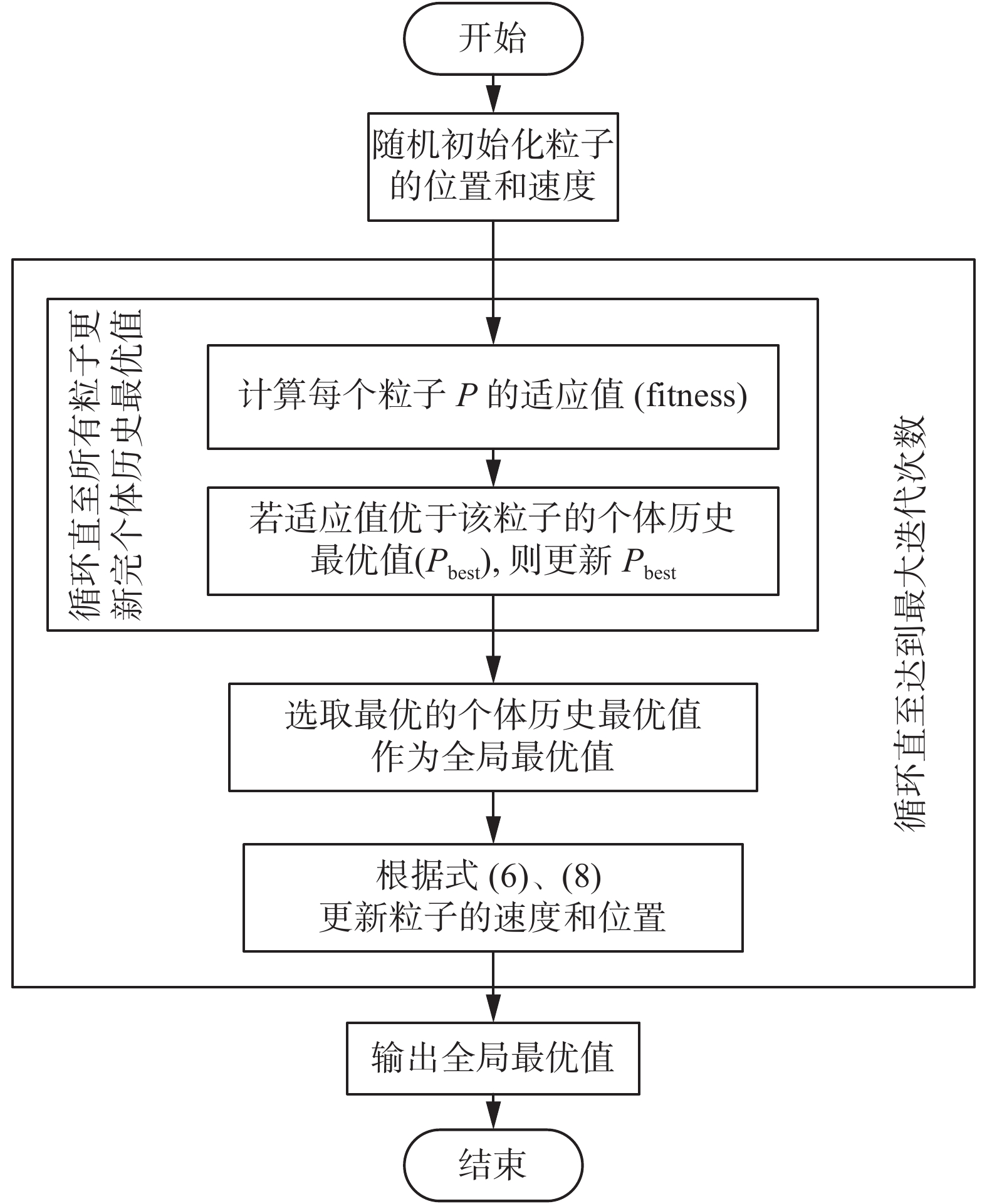Download: 图 2 CFPSO的流程 Fig. 2 Solution procedeure of the CFPSO
3 算例设计

3.1 算例1表 1 算例1代理商运输信息 Tab.1 Details of the transportation agency in case 1表 2 算例1城市间距离 Tab.2 Distances between cities in case 1
3.2 算例2表 3 算例2代理商运输信息 Tab.3 Details of the transportation agency in case 2表 4 算例2城市间距离 Tab.4 Distances between cities in case 2
3.3 算例3表 5 算例3城市间距离 Tab.5 Distances between cities in case 3
4 实验与结果分析 4.1 CFPSO参数设置 4.1.1 收敛因子k的确定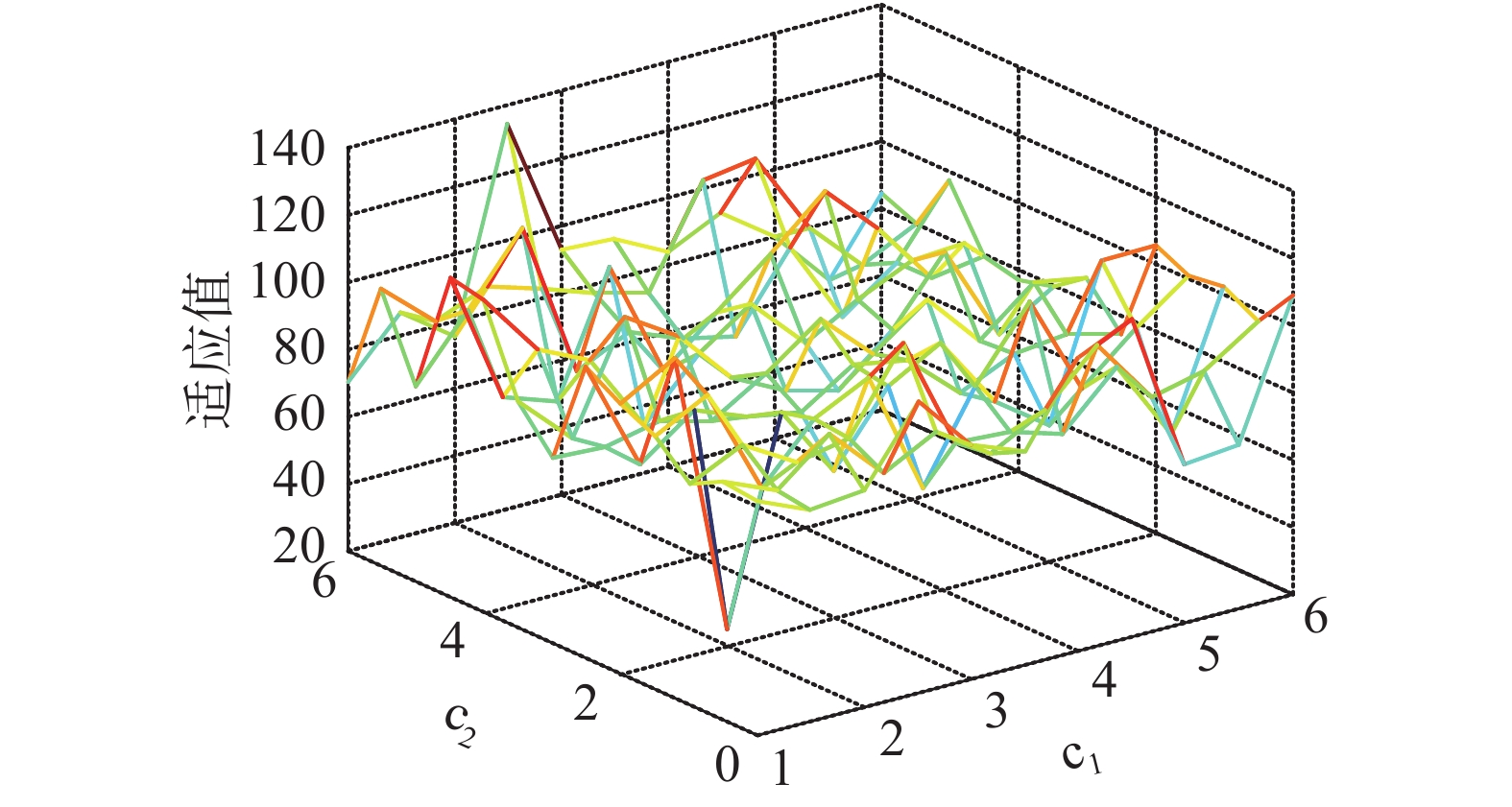Download: 图 3 学习因子对适应值的影响 Fig. 3 Influence of learning factors on fitness value表 6 适应值与 ${c_1}$ ${c_2}$ 的关系 Tab.6 Relationship between fitness values ${c_1}$ and ${c_2}$
4.1.2 隶属度函数中参数 $\beta$ 的确定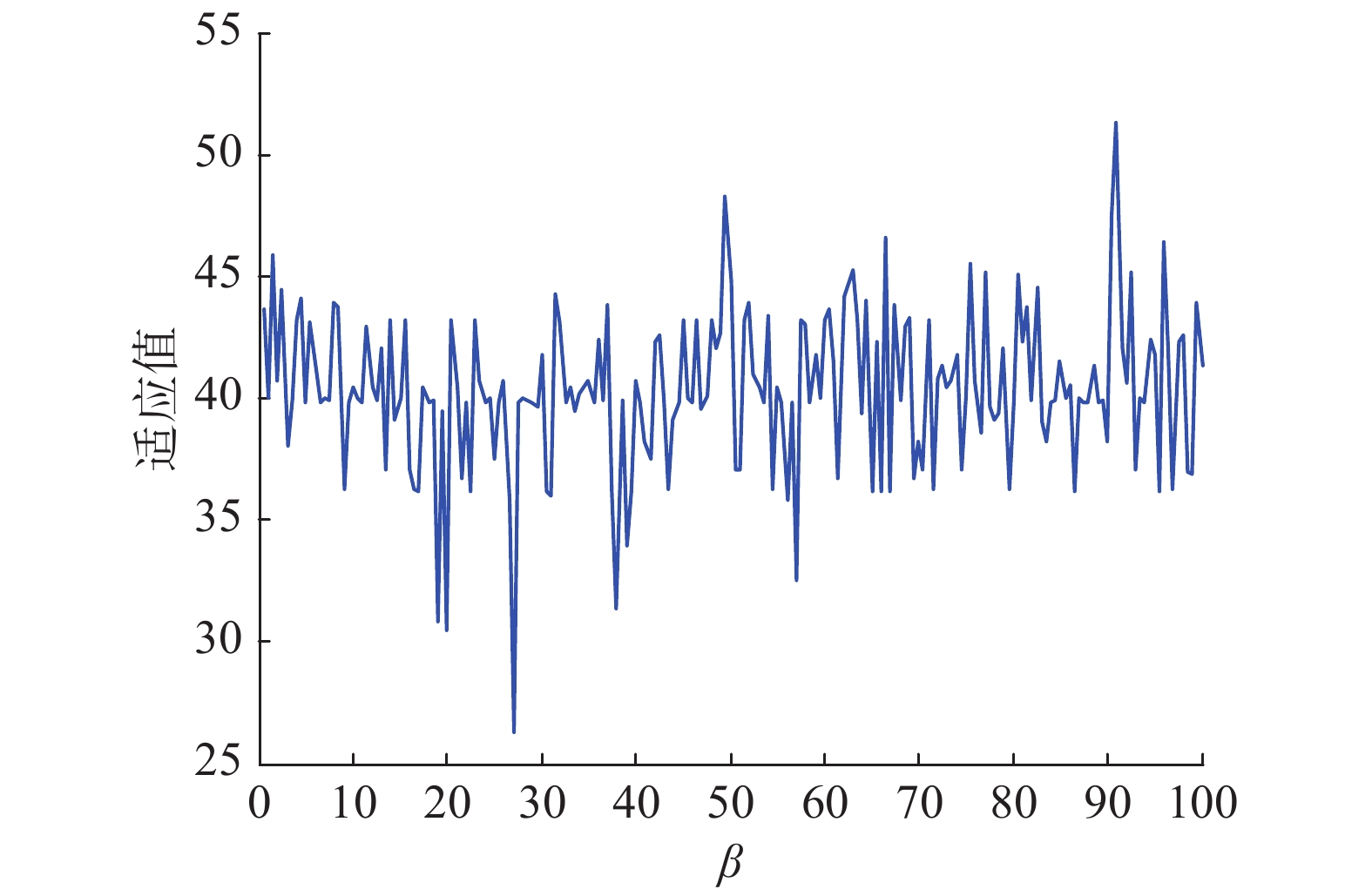Download: 图 4 参数 $\beta$ 对适应值的影响 Fig. 4 Influence of $\beta$ on fitness value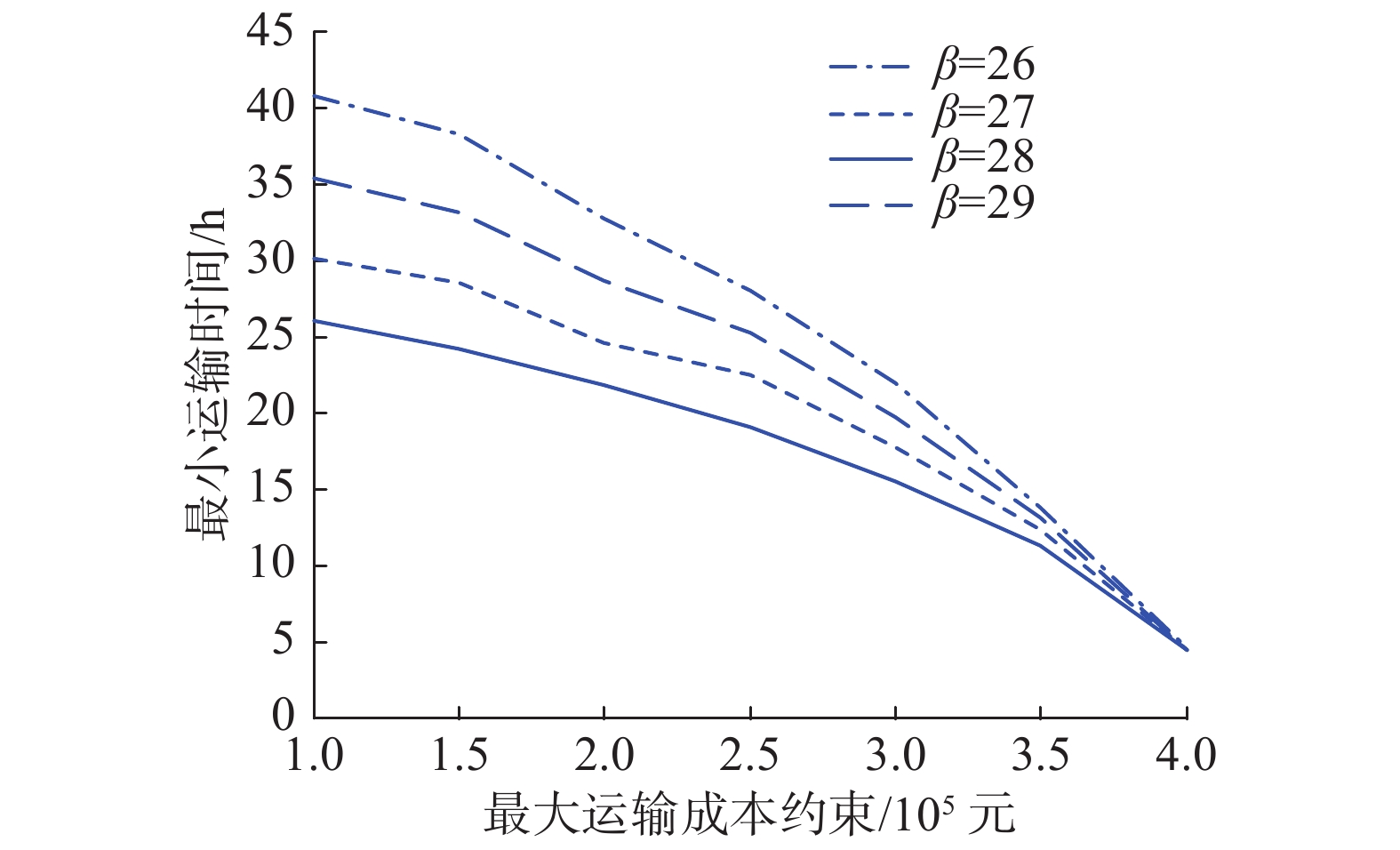Download: 图 5 参数 $\beta$ 对适应值的影响 Fig. 5 Influence of $\beta$ on fitness value

4.2 实验结果表 7 实验结果(算例3) Tab.7 Experimental results (case 3)
4.3 多种算法的对比分析表 8 各算法运行结果(算例1) Tab.8 Results obtained from the algorithm (case 1)表 9 各算法运行结果(算例2) Tab.9 Results obtained from the algorithm (case 2)表 10 各算法运行结果(算例3) Tab.10 Results obtained from the algorithm (case 3)

1)实验结果角度

2)CPU时间角度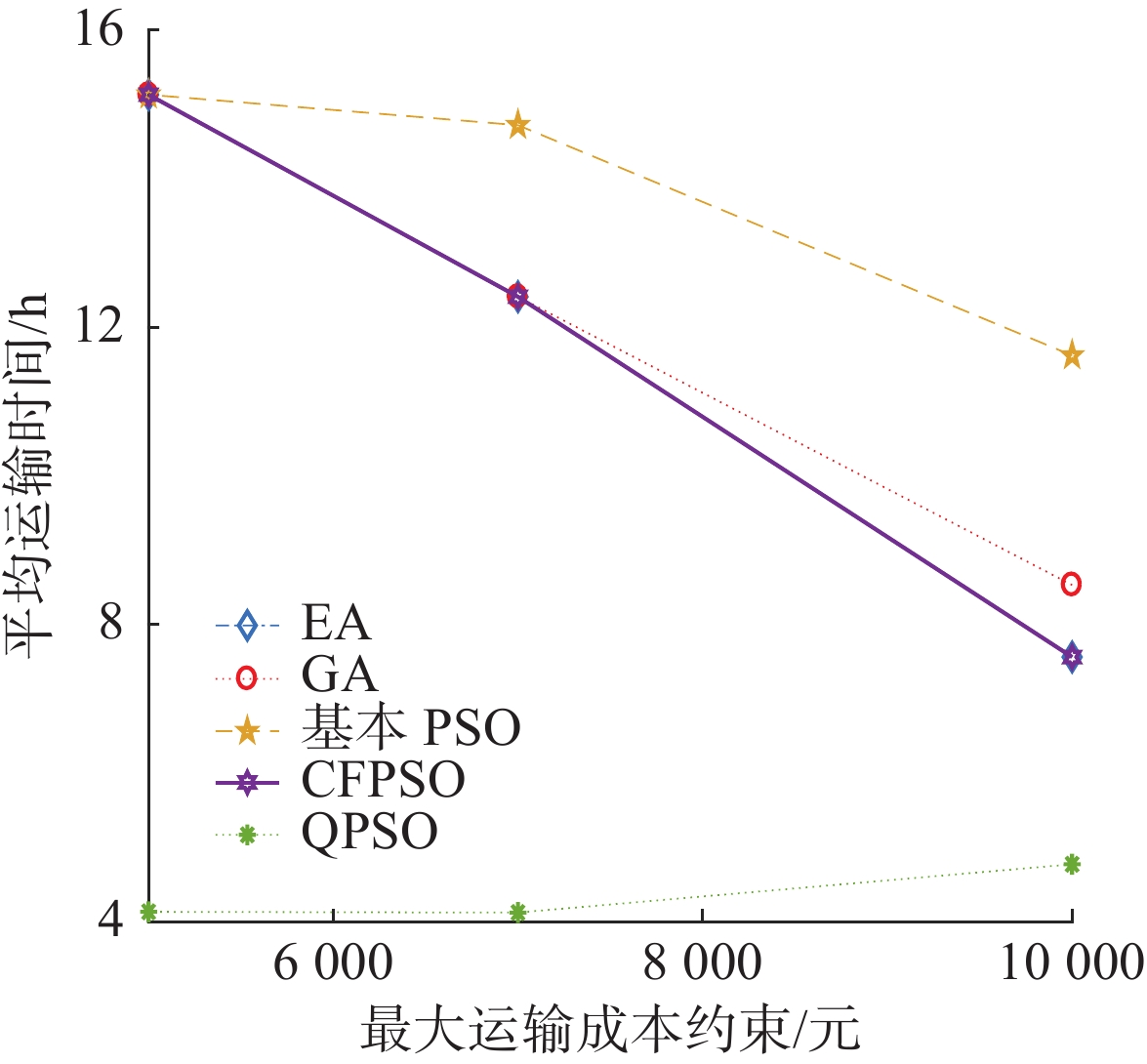Download: 图 6 算例1实验结果对比 Fig. 6 Comparison of experimental results in case 1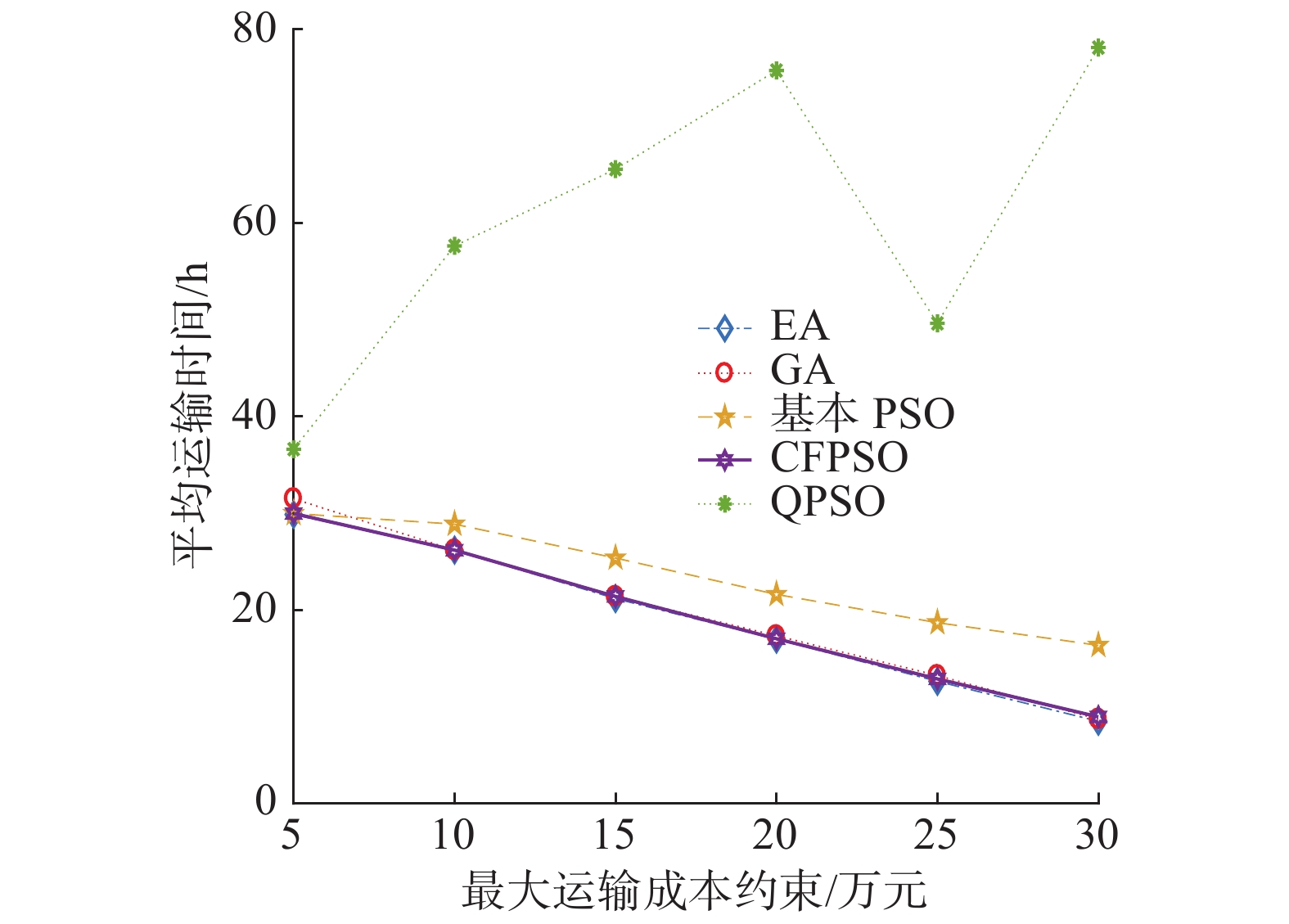Download: 图 7 算例2实验结果对比 Fig. 7 Comparison of experimental results in case 2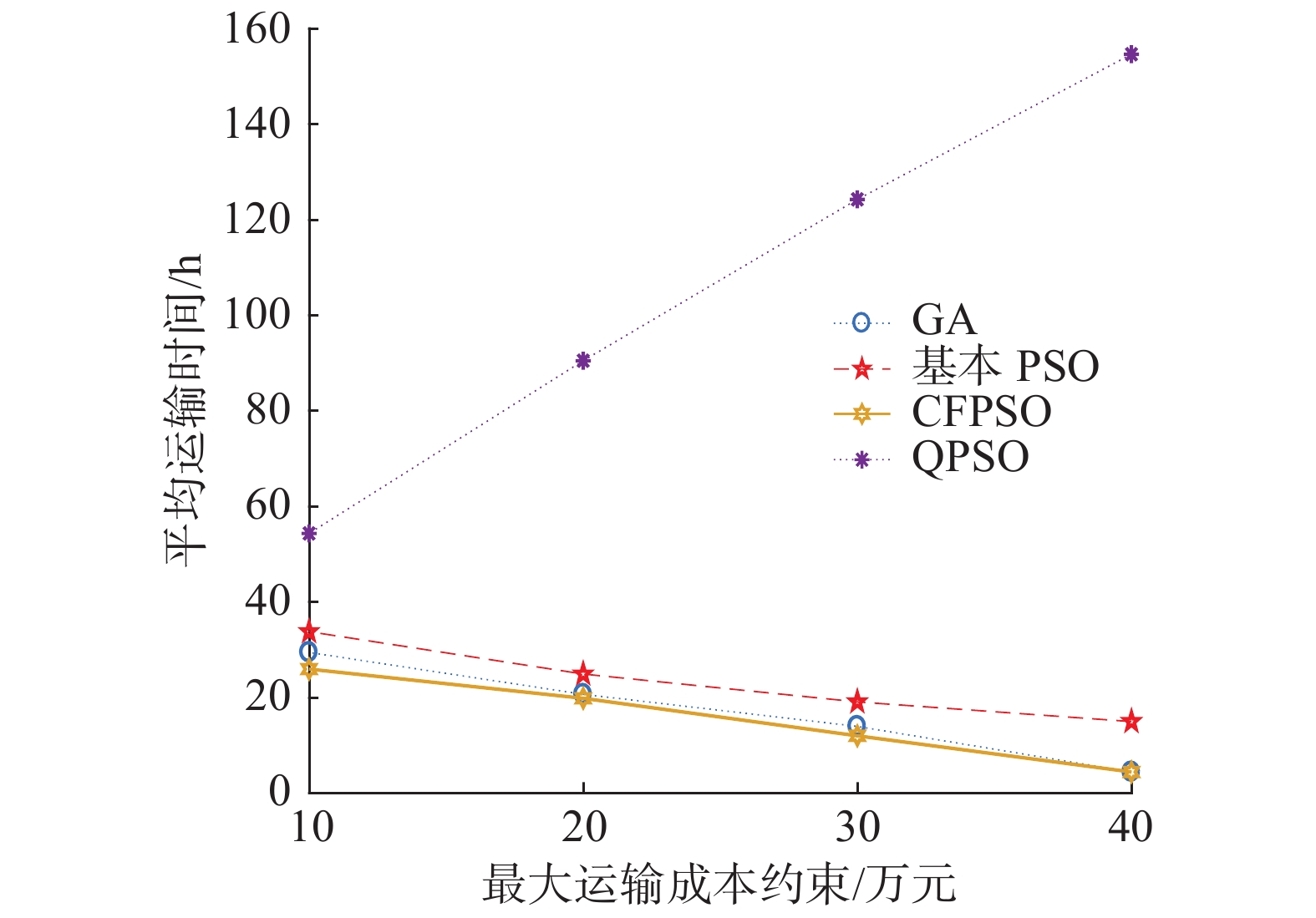Download: 图 8 算例3实验结果对比 Fig. 8 Comparison of experimental results in case 3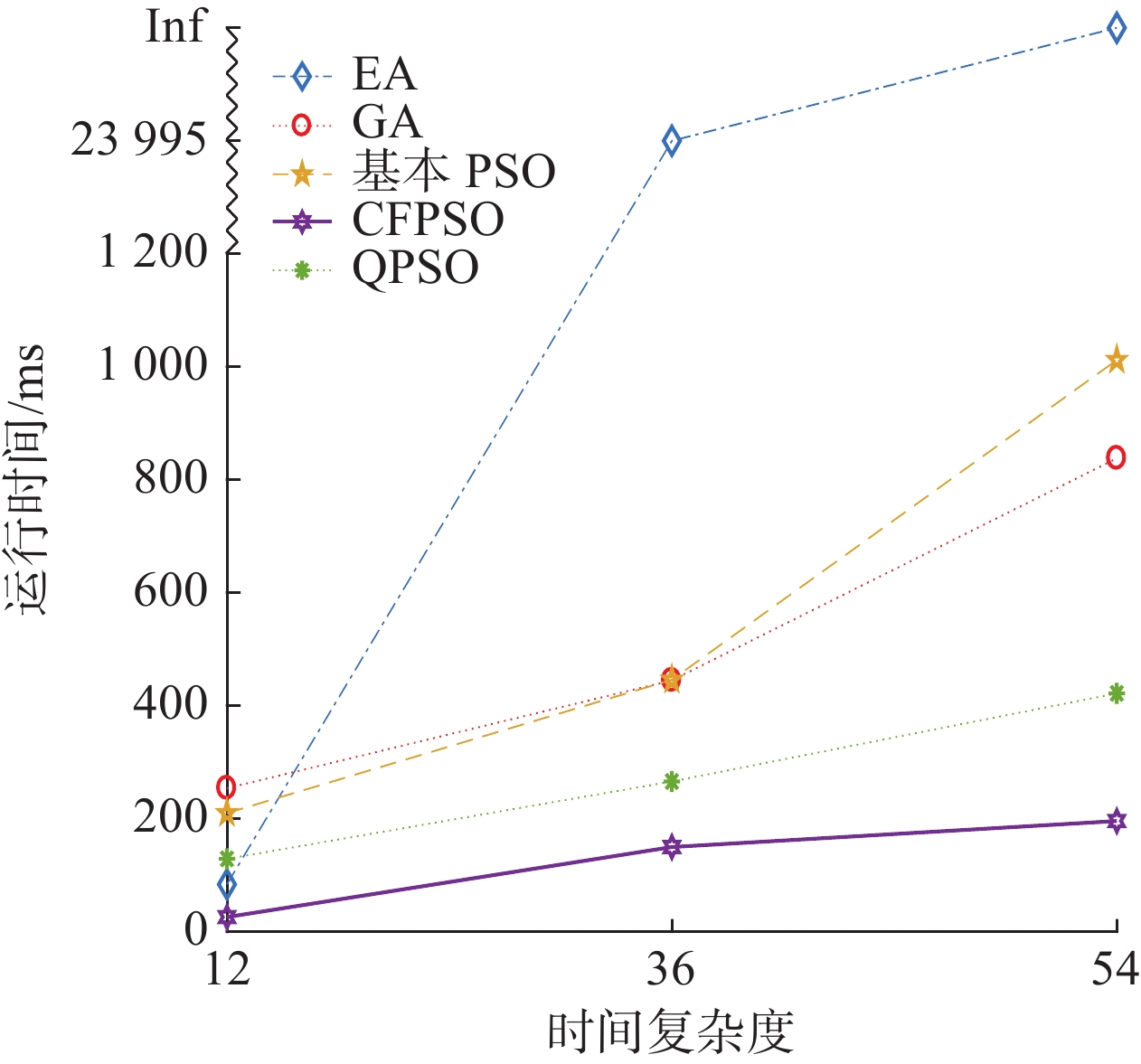Download: 图 9 各算法运行时间对比分析 Fig. 9 Comparison of the algorithm’s run time

5 结束语

  杨宝军, 李华增. 第四方物流剖析[J]. 工业工程与管理, 2003, 8(3): 49-51, 76. YANG Baojun, LI Huazeng. Analysis of the fourth party logistics[J]. Industrial engineering and management, 2003, 8(3): 49-51, 76. DOI:10.3969/j.issn.1007-5429.2003.03.012 (0)  姚建明, 刘丽文. 4PL模式下的供应链资源整合决策分析[J]. 系统工程, 2007, 25(4): 1-8. YAO Jianming, LIU Liwen. A decision analysis on supply Chain resource integration in 4PL mode[J]. Systems engineering, 2007, 25(4): 1-8. DOI:10.3969/j.issn.1001-4098.2007.04.001 (0)  BADE D J, MUELLER J K. New for the millenium -- 4PL[J]. Transportation & distribution, 1999, 40(2): 78-80. (0)  HUANG Min, TU Jun, CHAO Xiuli, et al. Quality risk in logistics outsourcing: a fourth party logistics perspective[J]. European journal of operational research, 2019, 276(3): 855-879. DOI:10.1016/j.ejor.2019.01.049 (0)  YAO Jianming. Decision optimization analysis on supply chain resource integration in fourth party logistics[J]. Journal of manufacturing systems, 2010, 29(4): 121-129. DOI:10.1016/j.jmsy.2010.12.002 (0)  张新, 田澎. 第四方物流及对物流规划功能的外包[J]. 工业工程与管理, 2002, 7(2): 38-40. ZHANG Xi, TIAN Peng. Fourth party logistics and outsourcing of planning function in logistics[J]. Industrial engineering and management, 2002, 7(2): 38-40. DOI:10.3969/j.issn.1007-5429.2002.02.009 (0)  HUANG Min, REN Liang, LEE L H, et al. Model and algorithm for 4PLRP with uncertain delivery time[J]. Information sciences, 2016, 330: 211-225. DOI:10.1016/j.ins.2015.10.030 (0)  崔妍, 黄敏, 王兴伟. 考虑中转发车时间4PLRP的模糊规划模型与算法[J]. 系统工程学报, 2012, 27(4): 535-542. CUI Yan, HUANG Min, WANG Xingwei. Fuzzy programming model and algorithm of 4PLRP considering travel schedule[J]. Journal of systems engineering, 2012, 27(4): 535-542. DOI:10.3969/j.issn.1000-5781.2012.04.015 (0)  薄桂华, 黄敏. 考虑拖期风险的第四方物流路径优化问题模型与求解[J]. 复杂系统与复杂性科学, 2018, 15(3): 66-74. BO Guihua, HUANG Min. Model and solution of routing optimization problem in the fourth party logistic eith tardiness risk[J]. Complex systems and complexity science, 2018, 15(3): 66-74. (0)  王勇, 赵骅, 李勇. 用禁忌算法求解第四方物流作业整合优化模型[J]. 系统工程学报, 2006, 21(2): 143-149. WANG Yong, ZHAO Hua, LI Yong. Tabu search algorithm for optimization model of integration of job of 4th party logistics[J]. Journal of systems engineering, 2006, 21(2): 143-149. DOI:10.3969/j.issn.1000-5781.2006.02.006 (0)  CUI Yan, HUANG Min, YANG Shengxiang, et al. Fourth party logistics routing problem model with fuzzy duration time and cost discount[J]. Knowledge-based systems, 2013, 50: 14-24. DOI:10.1016/j.knosys.2013.04.020 (0)  陈建清, 刘文煌, 李秀. 第四方物流中基于多维权的有向图模型及算法[J]. 工业工程与管理, 2003, 8(3): 45-48, 59. CHEN Jianqing, LIU Wenhuang, LI Xiu. The directed graph model with multi dimensions in the fourth party logistics and its algorithm[J]. Industrial engineering and management, 2003, 8(3): 45-48, 59. DOI:10.3969/j.issn.1007-5429.2003.03.011 (0)  KENNEDY J, EBERHART R C, SHI Y. Swarm intelligence[M]. San Francisco: Morgan Kaufmann Publishers, 2001: 1−10. (0)  SOMPRACHA C, JAYAWEERA D, TRICOLI P. Particle swarm optimisation technique to improve energy efficiency of doubly-fed induction generators for wind turbines[J]. The journal of engineering, 2019, 2019(18): 4890-4895. DOI:10.1049/joe.2018.9348 (0)  LAN Rushi, ZHU Yu, LU Huimin, et al. A two-phase learning-based swarm optimizer for large-scale optimization[J]. IEEE transactions on cybernetics, 2020, 2020:1−10. (0)  ABDELBAR A M, ABDELSHAHID S, WUNSCH II D C. Fuzzy PSO: A generation of particle swarm optimization[C]//Proceedings of International Joint Conference on Neural Networks. Montreal, Canada, 2005: 1086−1091. (0)  CHEN Chen, XU Junqi, LIN Guobin, et al. Fuzzy adaptive control particle swarm optimization based on T-S fuzzy model of maglev vehicle suspension system[J]. Journal of mechanical science and technology, 2020, 34(1): 43-54. DOI:10.1007/s12206-019-1247-4 (0)  伍永健, 陈跃东, 陈孟元. 量子粒子群优化下的RBPF-SLAM算法研究[J]. 智能系统学报, 2018, 13(5): 829-835. WU Yongjian, CHEN Yuedong, CHEN Mengyuan. Research on RBPF-SLAM algorithm based on quantum-behaved particle swarm optimization[J]. CAAI transactions on intelligent systems, 2018, 13(5): 829-835. (0)  GUANG He, LU Xiaoli. An improved QPSO algorithm and its application in fuzzy portfolio model with constraints[J]. Soft computing, 2021, 25(12): 7695-7706. DOI:10.1007/s00500-021-05688-3 (0)  ZHAO Xingang, LIANG Ji, MENG Jin, et al. An improved quantum particle swarm optimization algorithm for environmental economic dispatch[J]. Expert systems with applications, 2020, 152: 113370. DOI:10.1016/j.eswa.2020.113370 (0)  CHENG Jiatang, XIONG Yan, AI Li. Fault diagnosis of wind turbine gearbox based on neighborhood QPSO and improved D-S evidence theory[J]. Recent advances in computer science and communications, 2020, 13(2): 248-255. DOI:10.2174/2213275912666181218124805 (0)  CAI Yujie, SUN Jun, WANG Jie, et al. Optimizing the codon usage of synthetic gene with QPSO algorithm[J]. Journal of theoretical biology, 2008, 254(1): 123-127. DOI:10.1016/j.jtbi.2008.05.010 (0)  XU Xinzheng, SHAN Dong, WANG Guanying, et al. Multimodal medical image fusion using PCNN optimized by the QPSO algorithm[J]. Applied soft computing, 2016, 46: 588-595. DOI:10.1016/j.asoc.2016.03.028 (0)  LIN Sen, NAN Yurong. Optimization of rolling schedule in tandem cold mill based on QPSO algorithm[J]. Advanced materials research, 2010, 145: 165-170. DOI:10.4028/www.scientific.net/AMR.145.165 (0)  FAN Qiufeng, WANG Tao, CHEN Yang, et al. Design and application of interval Type-2 TSK fuzzy logic system based on QPSO algorithm[J]. International journal of fuzzy systems, 2018, 20(3): 835-846. DOI:10.1007/s40815-017-0357-3 (0)